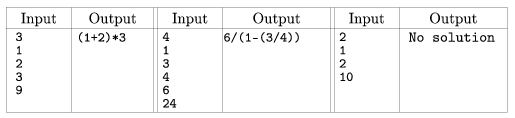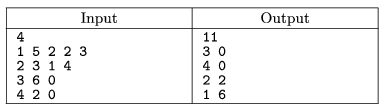Mathematical and Physical Journal
for High Schools
Issued by the MATFUND Foundation
 Already signed up? New to KöMaL?

# KöMaL Problems in Informatics, January 2006

Show/hide problems of signs:## Problems with sign 'I'

Deadline expired on February 15, 2006.

I. 121. We are given some positive integers a1,...,ak and an integer s. Write a program to decide whether the number s can be represented using the numbers ai (each of them exactly once), the four basic operations and some parentheses.

If there is a solution, print one, otherwise display No solution''.

The program should read from the keyboard (standard input). The first line contains the value of k (k6), the next k lines contain the numbers ai, while the (k+2)th line contains the prescribed result s.

Examples:The source code of the program (i121.pas, i121.cpp, ...) should be submitted.

(10 pont)

statistics

I. 122. Create a plain TeX file in which the formula for the solution of the quadratic equation is derived. Also investigate the number of real solutions. The beginning of the text should contain the usual KöMaL-header (number of the problem, name, city, class, school, e-mail address). The TeX source code (i122.tex) is to be submitted.

(10 pont)

solution (in Hungarian), statistics

I. 123. Create an OpenOffice or Excel sheet implementing the Euclidean algorithm to calculate the greatest common divisor of two positive integers with at most 6 digits.

The user enters the two integers into the first two cells of the first row. Every row then contains two numbers: the smaller number of the previous row and the remainder upon dividing the greater one by the smaller. These steps are repeated until a 0 appears among the numbers: the other number in that row is the greatest common divisor of the original numbers. This should be copied into the third cell of the first row.

See the example.

 12 21 3 12 9 9 3 3 0

The sheet (i123.sxc, i123.xls) should be submitted.

(10 pont)

solution (in Hungarian), statistics## Problems with sign 'S'

Deadline expired on February 15, 2006.

S. 14. The construction of a certain machine in a factory is divided into several phases. The number of minutes needed to complete a phase is known, together with the information which phases are required to precede that phase. Phases that are independent of one another can be performed simultaneously.

Make a schedule how to construct the machine so that the construction time is minimized, in a form that the starting minute for each phase is given.

The input consists of some positive integers encoding the phases. The first line contains the number of phases. Each subsequent line contains the name of a phase (also a number), its length (a positive integer), then the number of those phases on which the actual phase depends, finally their names separated by a space.

The first line of the output should contain the total construction time, then in each line the name of a phase together with its starting time, separated by a space.

See the example.The source code of the program (s14.pas, s14.cpp, ...) should be submitted.

(10 pont)

statistics

\$Var(Body)